Iodometry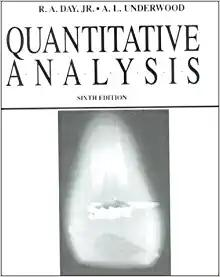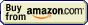Potentiometric titration » Curve calculation

As usual, there are three parts of the curve. Before equivalence point we assume reaction went to completion according to the reaction equation - and we use concentrations of both oxidized and reduced forms of titrated substance to calculate the potential. At equivalence point we use one of methods described on the potentiometric equivalence point calculation page. Finally after the equivalence point we assume that concentration of reacted form of the titrant is that given by the reaction stoichiometry and concentration of the unreacted form is that of added excess - and we use these concentrations in Nernst equation.

Example: what is potential in 0.01 M Fe3+ solution titrated 25% with 0.0112 M Ti3+? E0Fe3+/Fe2+ = 0.77 V, E0Ti4+/Ti3+ = 0.130 V.

This is reduction titration, in which Fe3+ is being reduced to Fe2+:

Fe3+ + Ti3+ → Fe2+ + Ti4+

Using approach described above we can immediately write1

as we know that already 25% of the iron is in the reduced form and so 100%-25%=75% have to be still in the oxidized form. We don't have to care about dilution factor, as it is identical for both forms of iron and cancels out. n is 1. Thus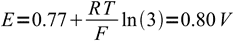2

Example: what is potential in 0.1 M H2O2 pH = 1.00 solution titrated 120% with 0.1 M permanganate? E0O2/H2O2 = 0.682 V, E0MnO4-/Mn2+ = 1.51 V.

In this titration hydrogen peroxide is oxidized by permanganate to water and oxygen:

5H2O2 + 2 MnO4- + 6H+ → 8H2O + 5O2 + 2 Mn2+

However, this reaction is irrelevant to the question. At 120% solution contains some amount of Mn2+ and 20% of that amount of excess permanganate. As both MnO4- and Mn2+ are present in the reaction quotient in the first power, their real concentrations don't matter, we are interested in the ratio only - and, similarly to the first question, we know enough to calculate this ratio as 20/100.

Nernst equation for the permanganate half reaction is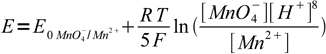3

From pH value we know H+ concentration is 0.1 M: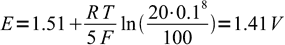4

Simplified method that we proposed above and used in the examples, is usually good enough, however, general method is not much harder. System is described by several equations - two Nernst equations describing half potentials of two redox systems involved, mass balances of both systems and stoichiometric equations describing dependencies between amounts of forms. Those stoichiometric equations follows reaction equation, so they are not hard to derive.

For example in the case of permanganometric determination of iron (II), reaction taking place is

5Fe2+ + MnO4- + 8H+ → 5Fe3+ + Mn2+ + 4H2O

It immediately follows that concentration of Fe3+ is five times higher than concentration of Mn2+. Sum of concentrations of both forms of iron is always identical (dilution ignored), sum of concentrations of permanganate and Mn2+ depends on the amount of titrant already added. Using all these information it is not difficult to derive equation - using approach similar to that used for calculation of equivalence point for iodine/thiosulfate titration, see potentiometric titration equivalence point calculation page - that allows exact calculation of the concentrations and redox potential. However, gain in accuracy in most cases doesn't justify effort required.